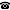# 2014 seminar talk: Maximal pseudocompactness and maximal countable compactness in the class of Tychonoff spaces

Talk held by Vladimir V. Tkachuk (Universidad Autónoma Metropolitana de México, Mexico City, Mexico) at the KGRC seminar on 2014-10-09.

### Abstract

This is a presentation of results obtained in 2013-2014 jointly with O.T. Alas and R.G. Wilson. Given a property $\mathcal P$, say that a space $X$ is maximal $\mathcal P$ in the class of Tychonoff spaces if $X$ has $\mathcal P$ but any stronger Tychonoff topology on $X$ does not have $\mathcal P$. It turns out that maximal pseudocompactness and maximal countable compactness in the class of Tychonoff spaces have more interesting properties than maximal pseudocompactness and maximal countable compactness in the class of all spaces so we will call the respective spaces maximal pseudocompact and maximal countably compact. Our presentation will include the following results (all spaces are assumed to be Tychonoff):

1) Any dyadic maximal pseudocompact space is metrizable.

2) Any Fréchet-Urysohn compact space is a retract of a Fréchet-Urysohn maximal pseudocompact space; since there are Fréchet-Urysohn compact spaces which are not maximal pseudocompact, maximal pseudocompactness is not preserved by continuous images even in compact spaces.

3) If $\kappa$ is strictly smaller than the first weakly inaccessible cardinal, then the Tychonoff cube $I^\kappa$ is maximal countably compact.

4) If $\lambda$ is the first measurable cardinal, then the Tychonoff cube $I^\lambda$ does not even embed in a maximal countably compact space.

5) If a space $X$ is maximal countably compact, then every $\omega$-continuous real-valued function on $X$ is continuous.

6) If $X$ is a countably compact space with the Mazur property, i.e., every sequentially continuous real-valued function on $X$ is continuous, then $X$ is maximal countably compact.

7) If $X$ is an $\omega$-monolithic compact space, then $C_p(X)$ has the Mazur property if and only if $C_p(X)$ is Fréchet-Urysohn.

Kurt Gödel Research Center for Mathematical Logic. Währinger Straße 25, 1090 Wien, Austria.+43-1-4277-50501. Last updated: 2010-12-16, 04:37.